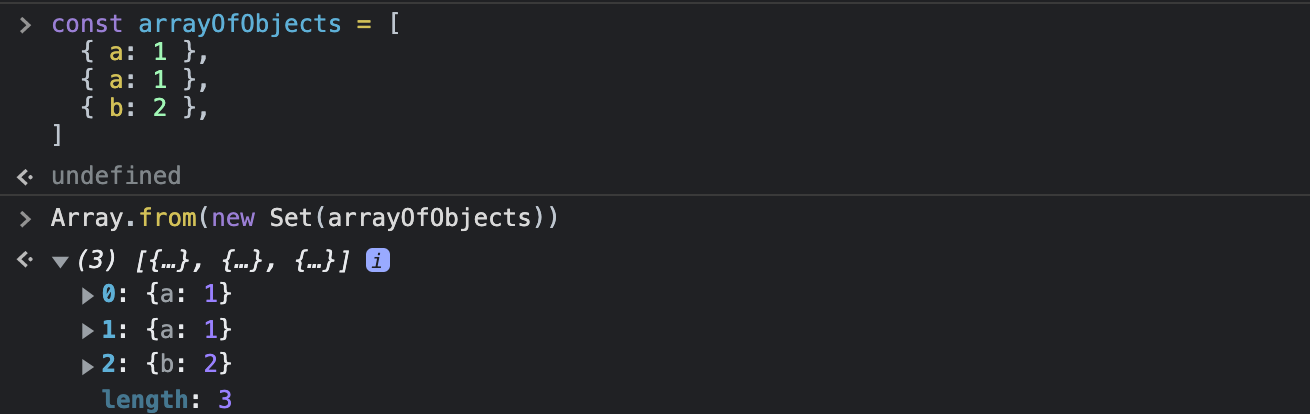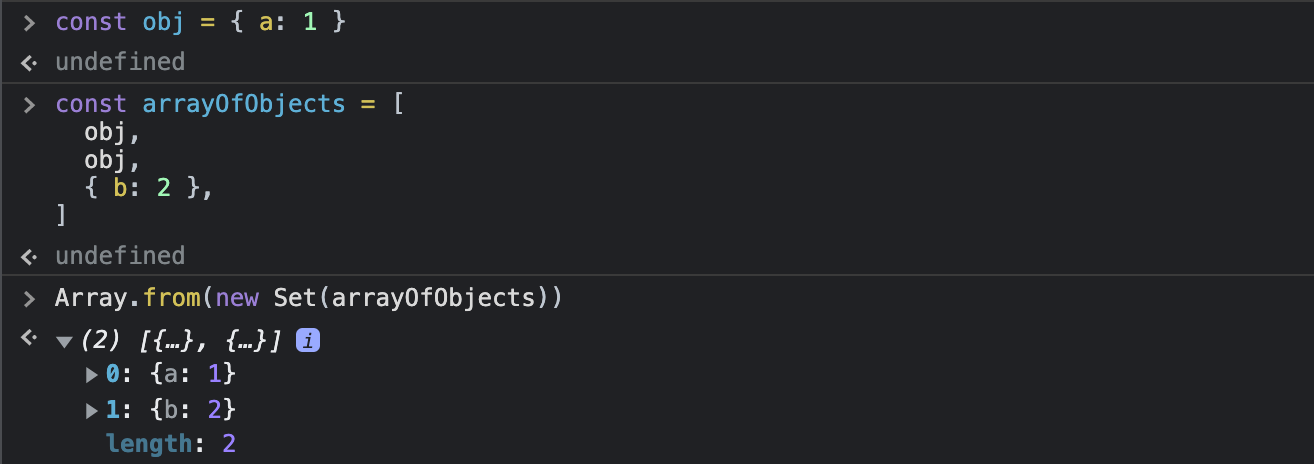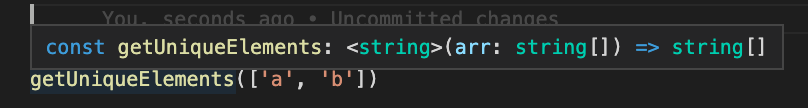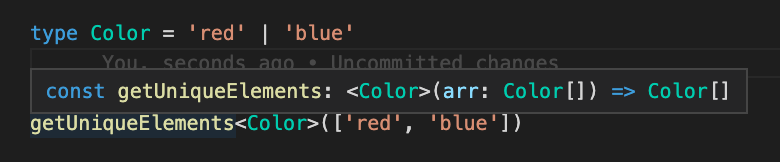# Remove duplicates from array in Javascript

2021-12-274 min## How to remove duplicates from array in Javascript / Typescript

In this article, I am going to show you how to get an array of unique elements using Set and Array.from method.

### Function creation

``````const getUniqueElements = (arr: any[]): any[] => Array.from(new Set(arr))
``````

First of all, we are creating a new Set from our array.

``````new Set(arr)
``````

Set is an object of unique values of any type. Set automatically remove duplicates.

The Set object lets you store unique values of any type, whether primitive values or object references. - From developer.mozilla.org

Second, we are creating an array from Set object by Array.from method. We can also use a spread operator instead.

``````Array.from(new Set(arr))
``````

or

``````[...new Set(arr)]
``````

### Usage examples

Let's take an example of an array of numbers (primitives).

``````[1, 2, 3, 4, 5, 1, 1, 2]
``````

So as you can see, there are repeated values 1 and 2. Our goal is to have an array of unique elements.

``````getUniqueElements([1, 2, 3, 4, 5, 1, 1, 2]) // output is [1, 2, 3, 4, 5]
``````

The same works for other primitives, but what about objects?

Well, with this method you can remove duplicated object references. You should know that sometimes, even if the objects looks the same, have equal keys and values, these might have different references.

If we create an array of objects this way:

``````const arrayOfObjects = [
{ a: 1 },
{ a: 1 },
{ b: 2 },
]
``````

then we have an array of three different objects. All of the objects have unique references, so all of the array elements are unique. Now if we use our function getUniqueElements(arrayOfObjects), the output will be:

``````[
{ a: 1 },
{ a: 1 },
{ b: 2 },
]
``````Now, let's create an object first

``````const obj = { a: 1 }
``````

and then create an array using our object reference (obj)

``````const arrayOfObjects = [
obj,
obj,
{ b: 2 },
]
``````

You probably noticed that I added our obj twice to the array. This means that we have duplicated object references in our array. Now if I use getUniqueElements(arrayOfObjects), the output will be:

``````[
{ a: 1 },
{ b: 2 },
]
``````And this way we removed duplicated object references from our array.

### Function typing

In the very beginning, I typed our function with an argument and the output as any[], but you can do it better. If you like to describe the array element type, you can use the generic type.

``````const getUniqueElements = <T extends any>(arr: T[]): T[] => Array.from(new Set(arr))
``````

Thanks to that, Typescript can infer the type, but you can also narrow the type passing the generic type.